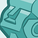# QlikView App Dev

Discussion Board for collaboration related to QlikView App Development.

cancel
Showing results for
Did you mean:Creator

## Aggregate Function Issue

Hi

All i have a question , i am currently have an expression which is doing a aggregate function over a variable and after that i am doing a calculation . I also have a cyclic group in the chart ( pivot table ) . The problem is aggregate function works for a certain selections and for certain selections it doesn't work (my thinking is   because the aggregated dimension has multiple values) and  the aggr function cannot understand . Is there any way to solve this

expression

if((aggr(\$(varibale1 ), dimension1, dimension 2, etc ))=0 or isnull((aggr(\$(variable 1),  dimension1, dimension 2 , etc))),

(SUM({\$<YYYY= {'2020'},>} \$(variable2 )))*(avg({\$<YYYY= {2020}=>} field ))

,\$(varibale1 ))

here the dimension in aggr function are same and dimenison2 falls into 2 categories of dimension 1 , so its failing to calculate the logic .

any help would be appreciated

2 RepliesCreator II

Hi, if you could provide some sample and the expected result, that would be helpful for others to help you. Thanks!Creator
Author

@alex00321 Attached is the image , the cyclic group and the dimension 2 values are added in the aggr . If you see the last two lines they are summing up but the first line is not summing up. The  expression used for first line

(SUM({\$<YYYY= {'2020'},>} \$(variable2 )))*(avg({\$<YYYY= {2020}=>} field )) because there is no new data for current year and its using previous year data  and multiplying with a field .